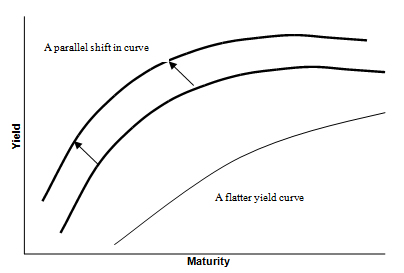# Yield Curve Risk

Updated September 16, 2020

## What is Yield Curve Risk?

Yield curve risk refers to the probability that the yield curve will shift in a manner that affects the values of securities tied to interest rates -- particularly, bonds.

## How Does Yield Curve Risk Work?

Also known as the term structure of interest rates, the yield curve is a graph that plots the yields of similar-quality bonds against their maturities, ranging from shortest to longest. (note that the chart does not plot coupon rates against a range of maturities -- that's called a spot curve.)

A yield curve can be positive (meaning the line goes up and to the right because investors require a higher rate of return for lending money for a longer period), inverted (meaning that the line slopes down and to the right), or flat. These shapes indicate not just whether short-term bond yields are higher or lower than long-term bond yields, but how investors feel about future interest rates and even economic growth.

Thus, if investors can look to the yield curve and see that certain maturities garner certain rates of return for a certain type of bond, they can build portfolios with the expectation that if interest rates change, their portfolios will react in a certain way.Any investor holding interest-rate-based securities is exposed to yield curve risk. Banks are particularly sensitive to changes in the yield curve. But predicting how the curve will change is tough. Most of the math is based on the assumption that the yield curve moves in a parallel manner (i.e., that all the yields for all the bonds change in the same way, causing the curve to keep its shape and simply move up or down). However, that is hardly ever what happens in the real world.

For example, sometimes the curve stays positive but it steepens, indicating that investors suddenly expect higher future inflation (and thus higher interest rates). Inversions of the curve tend to mean that investors now expect lower inflation (and interest rates) in the future. A flattening curve generally indicates investors are unsure about future economic growth. In turn, the investor carries yield curve risk--the risk that his portfolio won't change as planned when the yield curve changes.

## Why Does Yield Curve Risk Matter?

When interest rates in the market change, the price of a bond will change. There is an inverse relationship between price and yield: when bond prices go down, yields go up, and when bond prices go up, yields go down.

In addition to this price-yield relationship, there is an important relationship between yield and maturity. The yield curve is the graphical representation of that relationship in the market. If an investor or analyst is good at predicting changes in the yield curve, he or she will be able to benefit from the corresponding change in the prices of bonds.

Every bond portfolio has different exposures to how the yield curve shifts -- i.e. yield curve risk. The predicted percentage change in price of a bond that occurs when yields fluctuation is captured by an advanced concept called "duration."